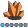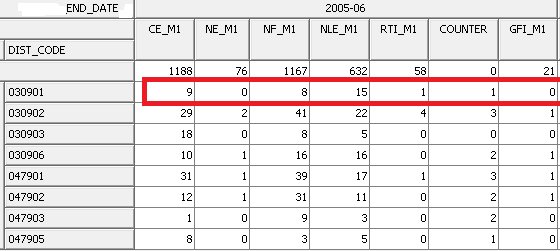SAPPER
Calcite | Level 5

## COMPARE multiple measure across a Hierachy/Dimension

Hello,

We have a cube that has six measures across multiple years and Districts as shown below.We are trying to mask the second highest value from any of the measures across every year * every district.

Example in the above table, lets take District = 030901 and we have the various measure values  (Disregard the COUNTER measure)  as

9 0 8 15 1 0

we need to mask 9 as . and the flags should be

. 0 8 15 1 0

The problem is traversing across multiple measures using MDX .

The SAS MDX documentation  talks about traversing down the hierarchies and dimensions and using a single measure .

Can anyone point me to a documentation /Example on how to traverse/get values for individual measures across the same dimension, i.e, traverse horizontally and not Top-Down ?

Thanks

SAPPERSAPPER
Calcite | Level 5

## Re: COMPARE multiple measure across a Hierachy/Dimension

One way we could accomplish this is to mask the data using pre-defined aggregation tables and then use them with the cube, but we really donot want to pre-define the aggregations and are looking at using the MDX.

Also on the OLAP cube it allows adding all the measures using MDX  as shown below in one of the calculated Members.

SUM(([DIMENSION_1].[HIERACHY_1],[DIMENSION_2].[HIERACHY_2]),GFI_M1+CE_M1+NE_M1+NLE_M1+RTI_M1+NF_M1 )

, FORMAT_STRING="BEST15.", SOLVE_ORDER=3

But the problem is comparing these multiple measures to get the max / min across dimensions on the Cube.

I used the SAS Information Map Studio to define a test measure which calculates the maximum of all the measures using MDX as

MAX((<<DIMENSION1.HIERACHY_1>>,<<DIMENSION2.HIERACHY_2>>), max(measures.members))

But that only gives me the maximum of all the measures used in the query, the problem now starts with how to loop across all the measures to compare their values with the above calculate maximum measure across the dimensions and hierarchies and to calculate the second largest value of all the measures..

Any Help would be greatly appreciated.

Thanks

SAPPER

Discussion stats